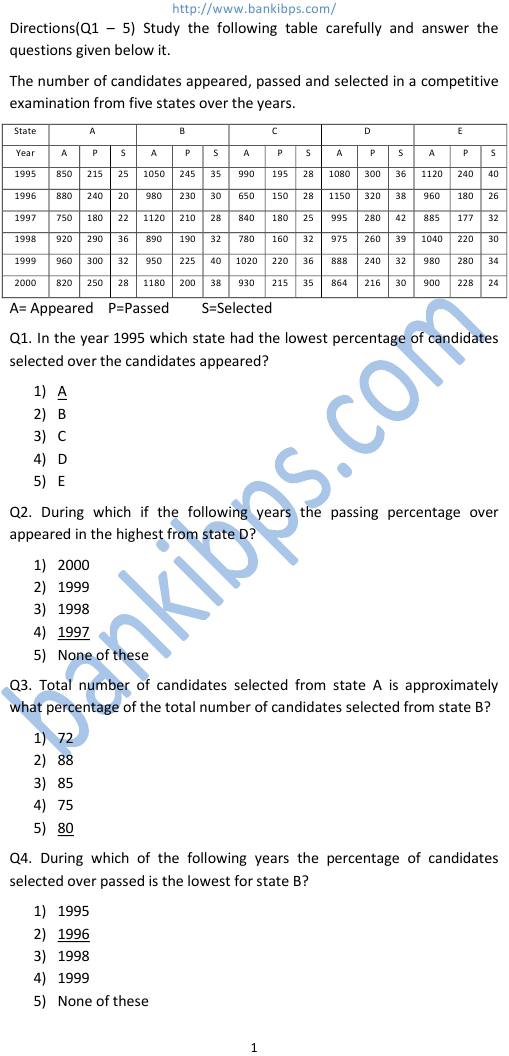# Data Analysis Questions for Bank Exams

Q1. In the year data analysis questions for bank exams 1995 which state had the lowest percentage of candidates selected over the candidates appeared? 1) A 2) B 3) C 4) D 5) E Q2. During which if the following years the passing percentage over appeared in the highest from state D? 1) 2000 2) 1999 3) 1998 4) 1997 5) None of these Q3. Total number of candidates selected from state A is approximately what percentage of the total number of candidates selected from state B? 1) 72 2) 88 3) 85 4) 75 5) 80 Q4. During which of the following years the percentage of candidates selected over passed is the lowest for state B? 1) 1995 2) 1996 3) 1998 4) 1999 5) None of these Q5. What is the ratio between number of candidates passed from state A in 1996 to that from state E in 1997? 1) 6:7 2) 14:15 3) 13:16 4) 12:16 5) None of these
Practice Exercise - 199 [ Data Interpretation ]## data analysis questions for bank exams

### test of data analysis and interpretation for bank exams

#### data analysis sample questions

##### question on data interpretation
###### data interpretation practice questions
test of data analysis and interpretation for bank exams. data analysis questions and answers. what is a data analysis. data analysis sample questions. data analysis test. data analysis practice. data analysis tests. data analysis guide. data analysis and interpretation questions and answers. question on data interpretation. data interpretation practice. data interpretation questions and answers. .data interpretation practice questions. data interpretation tests. data interpretation practice test. online data interpretation test. data interpretation sample questions. data interpretation online test.